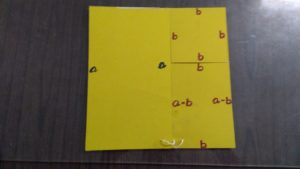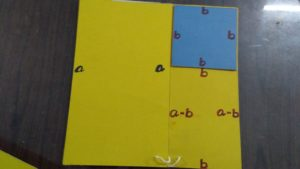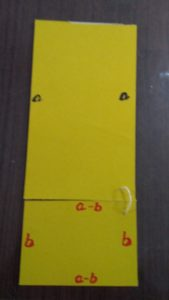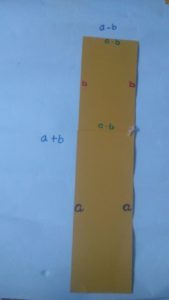# Hands On Training session with Mr.Ravi Aluganti

~ Kalai & Siva

In this session we learn about project plan that covers project definition, scope, schedule, resources, quality and budget . We gain practical experience and learn how to complete successful projects. We worked together as a team to complete a task of creating A TLM for Algebra formula in the most effective and efficient way. Hands-on tools make math a lot easier for young children to understand.

Equation:

a2-b2=(a + b)* (a + b)

ais the area of a square whose side is equal to a .

Now we have to subtract (remove) a square whose one side is equal to b from the above square.We cut the figure from here,And join this over here before joining turn it by 900This means a rectangle whose length is equal to (a + b) and breath is equal to (a-b)

Then the area of rectangle is l*b which means (a + b)* (a + b)

So here we get ,

a2-b2=(a + b)* (a + b)